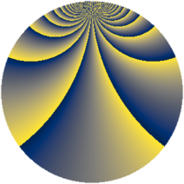# Properties

 Label 1140.2.cmLevel $1140$ Weight $2$ Character orbit 1140.cm Rep. character $\chi_{1140}(143,\cdot)$ Character field $\Q(\zeta_{36})$ Dimension $2784$ Sturm bound $480$

# Related objects

## Defining parameters

 Level: $$N$$ $$=$$ $$1140 = 2^{2} \cdot 3 \cdot 5 \cdot 19$$ Weight: $$k$$ $$=$$ $$2$$ Character orbit: $$[\chi]$$ $$=$$ 1140.cm (of order $$36$$ and degree $$12$$) Character conductor: $$\operatorname{cond}(\chi)$$ $$=$$ $$1140$$ Character field: $$\Q(\zeta_{36})$$ Sturm bound: $$480$$

## Dimensions

The following table gives the dimensions of various subspaces of $$M_{2}(1140, [\chi])$$.

Total New Old
Modular forms 2976 2976 0
Cusp forms 2784 2784 0
Eisenstein series 192 192 0

## Trace form

 $$2784q - 24q^{6} + O(q^{10})$$ $$2784q - 24q^{6} - 24q^{10} - 18q^{12} - 48q^{13} - 60q^{16} - 48q^{21} - 48q^{25} + 24q^{28} - 6q^{30} + 12q^{33} - 24q^{36} - 24q^{40} - 48q^{42} - 12q^{45} - 72q^{46} + 72q^{48} - 24q^{52} - 24q^{57} + 96q^{58} - 12q^{60} - 96q^{61} + 48q^{66} - 24q^{70} - 60q^{72} - 48q^{73} + 24q^{76} + 30q^{78} - 48q^{81} - 312q^{82} - 48q^{85} - 36q^{88} - 12q^{90} + 12q^{93} - 144q^{96} - 48q^{97} + O(q^{100})$$

## Decomposition of $$S_{2}^{\mathrm{new}}(1140, [\chi])$$ into newform subspaces

The newforms in this space have not yet been added to the LMFDB.